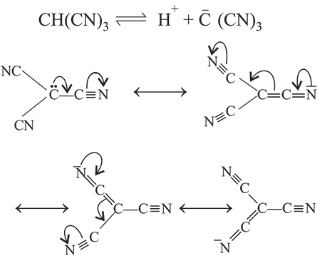# Which amongst the following is the strongest acid?Question:

Which amongst the following is the strongest acid?

1. $\mathrm{CHBr}_{3}$

2. $\mathrm{CH}(\mathrm{CN})_{3}$

3. $\mathrm{CHI}_{3}$

4. $\mathrm{CHCl}_{3}$

Correct Option: , 3

Solution:

Due to the resonance stabilisation of the conjugate base, $\mathrm{CH}(\mathrm{CN})_{3}$ is the strongest acid amongst the given compounds.The conjugate bases of $\mathrm{CHBr}_{3}$ and $\mathrm{CHl}_{3}$ are stabilised by inductive effect of halogens. This is why, they are less stable. Also, the conjugate base of $\mathrm{CHCl}_{3}$ involves back-bonding between $2 p$ and $3 p$ orbitals